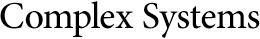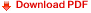## The Slowdown Theorem: A Lower Bound for Computational Irreducibility in Physical SystemsJonathan Gorard
Department of Mathematics, King’s College London
Algorithms R&D Group, Wolfram Research, Inc.
jonathan.gorard@kcl.ac.uk

#### Abstract

Following from the work of Beggs and Tucker on the computational complexity of physical oracles, a simple diagonalization argument is presented to show that generic physical systems, consisting of a Turing machine and a deterministic physical oracle, permit computational irreducibility. To illustrate this general result, a specific analysis is provided for such a system (namely a scatter machine experiment (SME) in which a classical particle is scattered by a sharp wedge) and proves that it must be computationally irreducible. Finally, some philosophical implications of these results are discussed; in particular, it is shown that the slowdown theorem implies the existence of classical physics experiments with undecidable observables, as well as the existence of a definite lower bound for the computational irreducibility of the laws of physics. Therefore, it is argued that the hypothesis that “the universe is a computer simulation” has no predictive (i.e., only retrodictive) power.

Keywords: computational irreducibility; computational equivalence; computational complexity; Turing machines; physics; simulations;  slowdown; experiments